# Electronics and Communication Engineering - Exam Questions Papers

36.

Find the value of ix if each resistance is of 3 Ω and each battery is of 6 V.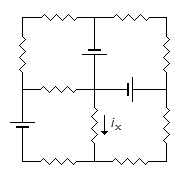A. 1.1428 V B. 1.432 A C. 0.2892 A D. O A

Explanation:

Using Mesh analysis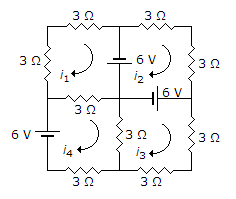Loop 1 : 6 = 9i1 - 3i4 ...(i)

Loop 2: 6 + 6 = - 6i2

i2 = - 2A ...(ii)

Loop 3: 6 = 9i3 - 3i4 ...(iii)

Loop 4: 6 = 9i4 - 3i3 - 3i1 ...(iv)

Solving the above equations simultaneously

i1 = i3 = 1.1428 A

i4 = 1.432 A

ix = i4 - i3 = 0.2892 A.

37.

An image uses 512 x 512 picture elements. Each of the picture elements can take any of the 8 distinguishable intensity levels. The maximum entropy in the above image will be

 A. 2097152 bits B. 786432 bits C. 648 bits D. 144 bits

Explanation:

Picture elements (pixel) = 512 x 512 = 262144

Information per picture element N = log2 M

M = 2N

8 = 2N

N = 3

Information for 262144 pixels = 262144 x 3 = 786432 bits

Entropy is same as average information per message.

38.

Find RTH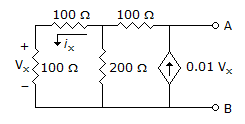A. 200 Ω B. 0 Ω C. 400 Ω D. None of these

Explanation:

Since there is no independent source in the network

VTH = O V

To find RTh apply : A current source of 1 A across A and B.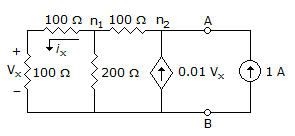Apply KCL at node n1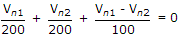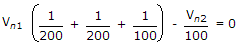Apply KCL at node Vn2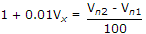Vn1 = 2Vx put in (i)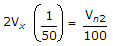Vn2 = 4vx

put in (ii)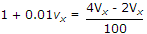100 + Vx = 2Vx

100 = Vx

Vn2 = 4Vx = 400 V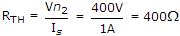.

39.

If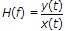then for this to be true x(t) is __________ .

 A. exp (j2pf/t) B. exp (-j2pf/t) C. exp (j2pft) D. exp (-j2pft)

Explanation:

Consider x(t) = ej2pft

y(t) = h(t) * x (t)

y(t) =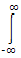h(t). ej2pf(t - t) . dt

= ei2pfth(t) . e- j2pft . dt

= ej2pft x H(f) = x(t) x H(f)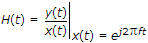.

40.

The dual transform of the given network as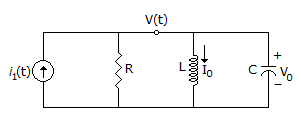A.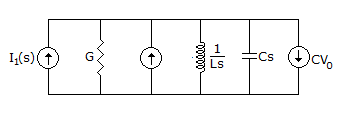B.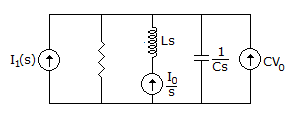C.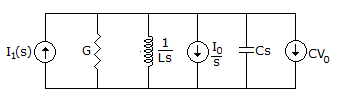D. none of the above

Explanation:

Dual of R → G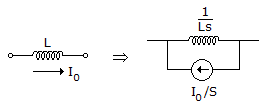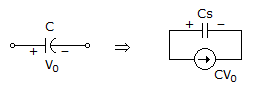#### Current Affairs 2022

Interview Questions and Answers